# Eigenvalues Calculator

Select the dimension of matrix, input the values, and press calculate button to find the eigenvalues

Give Us Feedback

## Eigenvalue Calculator

Solve matrices of different dimensions from 2x2 to 6x6 for eigenvalues and eigenvectors using this calculator. The calculations section consists of procedures to find each and every value for the respective matrix.

## What are eigenvalues and eigenvectors?

After transformation, a function applied in 2 or more dimensions, the vector which satisfies the equation Av = λv is called eigenvectors (v).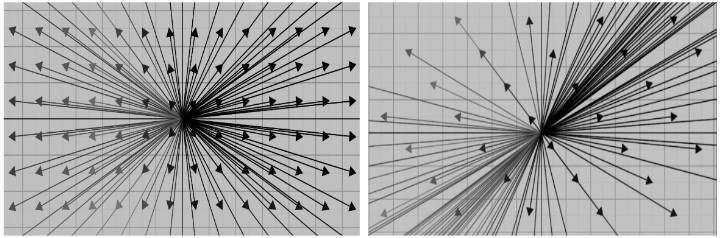This equation is called eigenequation. It describes how a certain set of vectors that are multiplied with a scalar quantity like 2 or 7 e.t.c are equal to a transformation matrix multiplied by a vector.

The scalar quantity in the equation is called the eigenvalue (λ). This equation is a little strange when one thinks about how a matrix-vector multiplication is equal to scalar-vector multiplication but it is true nonetheless.

The transformation matrix (A) is made by placing two or more vectors column-vise that represent the basic vectors like i, j, and k after transformation.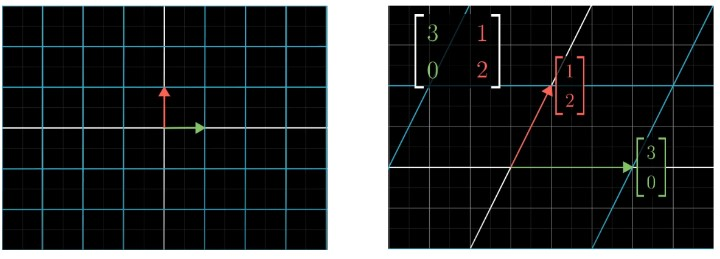In the image above, the i (along the x-axis) vector stretched by 3 times “along its span”. This made it an eigenvector as well. While the second basic vector j (along the y-axis) stretched and changed its direction from its span.

The eigenvectors are such vectors in a plane that during the movement or transformation stay on their span that passes through it. These vectors may stretch or compress depending on the transformation.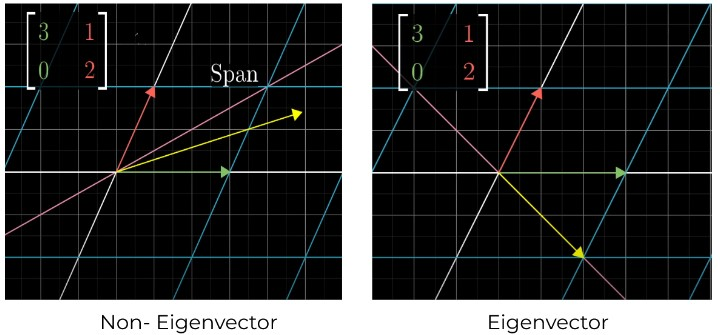This is why working with eigenspaces, the span of an eigenvector, is more suitable. Because any vector along that span will satisfy the equation Av = λv

The maximum number of eigenvectors depends on the size of the transformation matrix. If it is 3x3, the maximum number of such vectors will be 3. Though it is possible to have fewer, one, or no eigenvectors.

## How to find eigenvectors and eigenvalues?

The same eigenequation is modified for this purpose. The goal is to make both slides some version of matrix-vector multiplication.

One way to do that is to multiply the eigenvalue with an identity matrix since it makes no change as such.

Av = λIv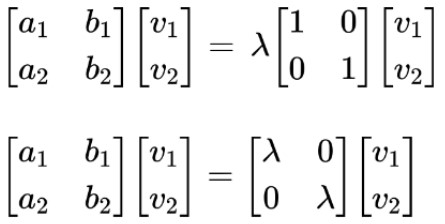This is now the desired form. Rearranging it:

Av - λIv = 0

(A - λI)v = 0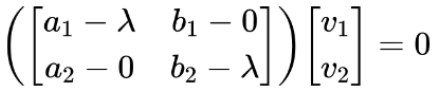The vector is taken as common. The value (A - λI) after solving is still a matrix. This equation is true for eigenvectors if and only if the product of this matrix and vector is zero.

If the dimensions of a vector are reduced by 1, the result will be zero. But how to do that? When the determinant of a matrix is zero, that means the vector has shifted to a lower dimension.

det(A - λI) = 0

This means we have to find a special value for lambda or the eigenvalue that will make the determinant zero. And that in turn will be used to calculate the eigenvector.

Eigenvalues are calculated by solving the mentioned equation. The end result is usually a polynomial equation that is broken down for roots i.e eigenvalues.

Eigenvectors will be calculated when these eigenvalues are used in the eigenequation and reduced using the Gaussian Elimination method.

### Example:

What are the eigenvalues and eigenvectors for the following matrix?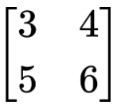Solution:

Finding eigenvalues:

Step 1: Put the matrix in the formula.

det(A - λI) = 0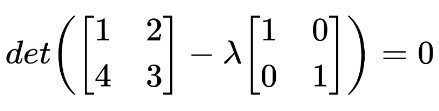Step 2: Multiply the lambda and subtract with the matrix A.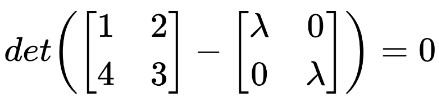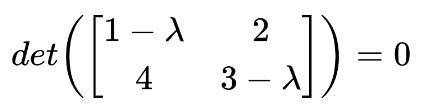Step 3: Take the determinant.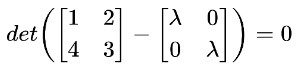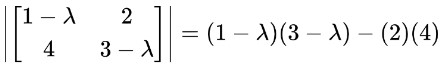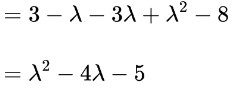Step 4: Break down into roots using the Quadratic formula calculator.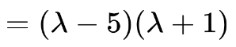Hence, the eigenvalues are λ = 5 and λ = -10

Finding the eigenvectors:

Step 1: Put the first value of lambda in the matrix.

(A - λI)v = 0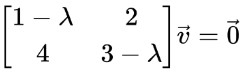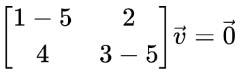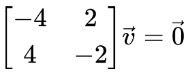Step 2: Perform row echelon reduction.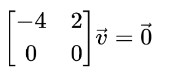1. Divide R1 by -4.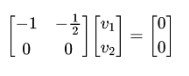Step 3: Translate back to a matrix equation, the reduced system is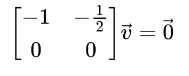As a scalar equation, this system is:

v1 - v2 / 2 = 0

v1 = v2 / 2

According to the above equation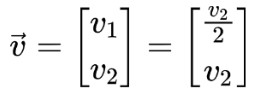Letting v2 = 1, the first eigenvector we get is: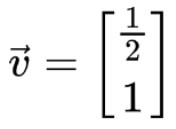Step 4: Similarly, find the second eigenvector.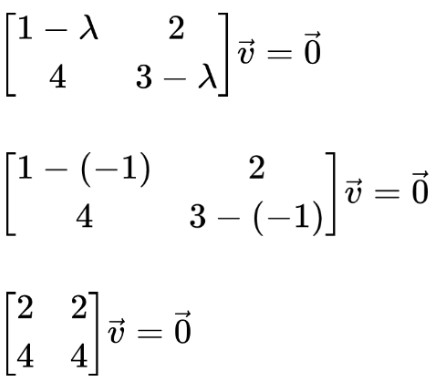Swap R1 with R2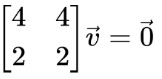Multiply ½ with R1 and subtract from R2.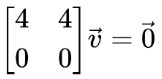Divide R1 by 4.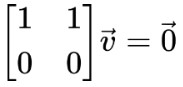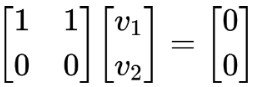As a scalar equation, this system is:

v1 + v2 = 0

v1 = -v2

According to the above equation: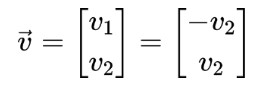Letting v2 = 1, the second eigenvector we get is: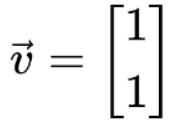### Math Tools